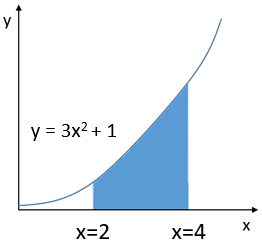## A Curve’s Area

What is the area of the shaded region in the graph shown? Note the figure is not to scale.Hint
Solve the definite integral of the slope while setting the lower and upper bound limits to determine the specified area under the curve.
Hint 2
The power rule:
$$\int x^n \:dx=\frac{x^{n+1}}{n+1}$$$Solving the definite integral of the slope while setting the upper and lower bound limits will determine the specified area under the curve. $$A=\int_{2}^{4}3x^{2}+1\:dx$$$
Recall the power rule:
$$\int x^n \:dx=\frac{x^{n+1}}{n+1}$$$Thus, $$A=\frac{3}{3}x^{2+1}+1x\bigg\rvert_{x=2}^{x=4}=x^{3}+x\bigg\rvert_{x=2}^{x=4}$$$
$$=4^{3}+4-[2^{3}+2]=64+4-[8+2]=68-10=58$$\$
58